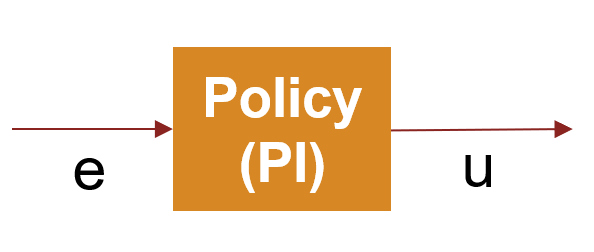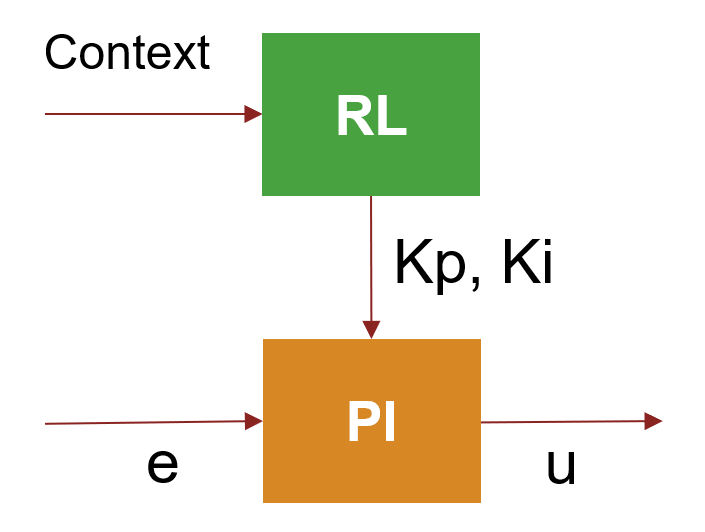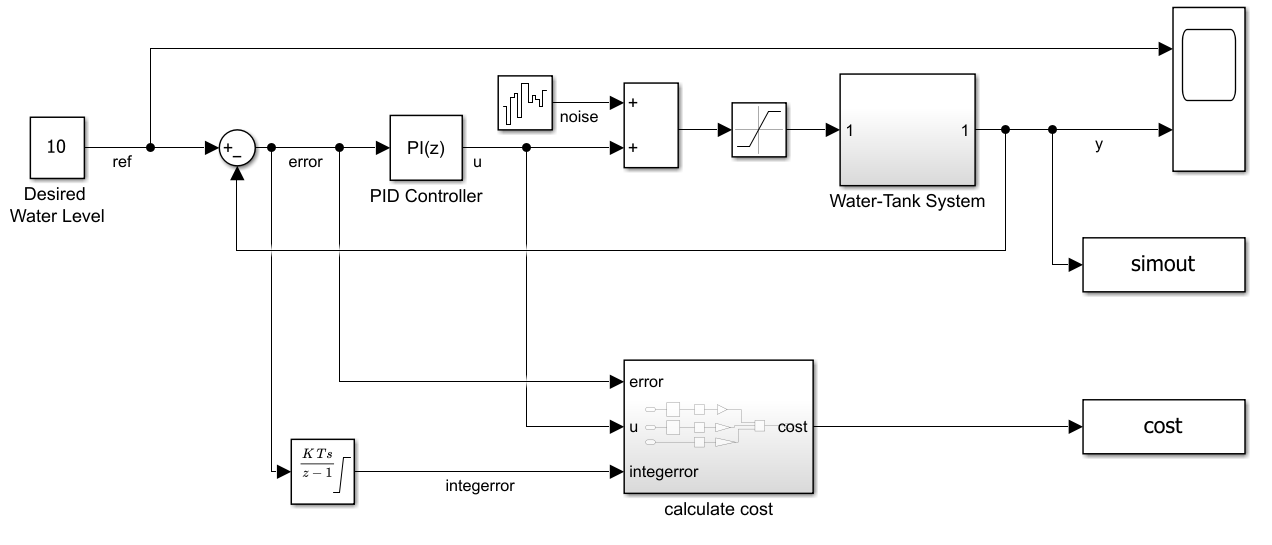# Tune PI Controller using Reinforcement Learning

This example shows how to tune a PI controller using the twin-delayed deep deterministic policy gradient (TD3) reinforcement learning algorithm. The performance of the tuned controller is compared with that of a controller tuned using the Control System Tuner app. Using the Control System Tuner app to tune controllers in Simulink® requires Simulink Control Design™ software.

For relatively simple control tasks with a small number of tunable parameters, model-based tuning techniques can get good results with a faster tuning process compared to model-free RL-based methods. However, RL methods can be more suitable for highly nonlinear systems or adaptive controller tuning.

To facilitate the controller comparison, both tuning methods use a linear quadratic Gaussian (LQG) objective function.

This example uses a reinforcement learning (RL) agent to compute the gains for a PI controller. For an example that replaces the PI controller with a neural network controller, see Create Simulink Environment and Train Agent.

### Environment Model

The environment model for this example is a water tank model. The goal of this control system is to maintain the level of water in a tank to match a reference value.

`open_system('watertankLQG')`The model includes process noise with variance $\mathit{E}\left({\mathit{n}}^{2}\left(\mathit{t}\right)\right)=1$.

To maintain the water level while minimizing control effort `u`, the controllers in this example use the following LQG criterion.

`$\mathit{J}=\underset{\mathit{T}⇒\infty }{\mathrm{lim}}\mathit{E}\left(\frac{1}{\mathit{T}}{\int }_{0}^{\mathit{T}}\left({\left(\mathit{Href}-\mathit{y}\left(\mathit{t}\right)\right)}^{2}+0.01{\mathit{u}}^{2}\left(\mathit{t}\right)\right)\mathrm{dt}\right)$`

To simulate the controller in this model, you must specify the simulation time `Tf` and the controller sample time `Ts` in seconds.

```Ts = 0.1; Tf = 10;```

### Tune PI Controller using Control System Tuner

To tune a controller in Simulink using Control System Tuner, you must specify the controller block as a tuned block and define the goals for the tuning process. For more information on using Control System Tuner, see Tune a Control System Using Control System Tuner (Simulink Control Design).

For this example, open the saved session `ControlSystemTunerSession.mat` using Control System Tuner. This session specifies the PID Controller block in the `watertankLQG` model as a tuned block and contains an LQG tuning goal.

```controlSystemTuner("ControlSystemTunerSession") ```

To tune the controller, on the Tuning tab, click Tune.

The tuned proportional and integral gains are approximately 9.8 and 1e-6, respectively.

```Kp_CST = 9.80199999804512; Ki_CST = 1.00019996230706e-06;```

### Create Environment for Training Agent

To define the model for training the RL agent, modify the water tank model using the following steps.

1. Delete the PID Controller.

2. Insert an RL Agent block.

3. Create the observation vector ${\left[\begin{array}{ccc}\int \mathit{e}\text{\hspace{0.17em}}\mathrm{dt}& \mathit{e}& \end{array}\right]}^{\mathit{T}\text{\hspace{0.17em}}}$ where $\mathit{e}=\mathit{Href}-\mathit{h}$, $\mathit{h}$ is the height of the tank, and $\mathit{Href}$ is the reference height. Connect the observation signal to the RL Agent block.

4. Define the reward function for the RL agent as the negative of the LQG cost , that is, $\mathrm{Reward}=-\left({\left(\mathit{Href}-\mathit{h}\left(\mathit{t}\right)\right)}^{2}+0.01{\mathit{u}}^{2}\left(\mathit{t}\right)\right)$. The RL agent maximizes this reward, thus minimizing the LQG cost.

The resulting model is `rlwatertankPIDTune.slx`.

```mdl = 'rlwatertankPIDTune'; open_system(mdl)```Create the environment interface object. To do so, use the `localCreatePIDEnv` function defined at the end of this example.

`[env,obsInfo,actInfo] = localCreatePIDEnv(mdl);`

Extract the observation and action dimensions for this environment.

```numObservations = obsInfo.Dimension(1); numActions = prod(actInfo.Dimension);```

Fix the random generator seed for reproducibility.

`rng(0)`

#### Create TD3 Agent

Given observations, a TD3 agent decides which action to take using an actor representation. To create the actor, first create a deep neural network with the observation input and the action output. For more information, see `rlContinuousDeterministicActor`.

You can model a PI controller as a neural network with one fully-connected layer with error and error integral observations.

`$\mathit{u}=\text{\hspace{0.17em}}\left[\begin{array}{ccc}\int \mathit{e}\text{\hspace{0.17em}}\mathrm{dt}& \mathit{e}& \end{array}\right]*{\left[\begin{array}{ccc}{\mathit{K}}_{\mathit{i}}& {\mathit{K}}_{\mathit{p}}& \end{array}\right]}^{\mathit{T}\text{\hspace{0.17em}}}$`

Here:

• `u` is the output of the actor neural network.

• `Kp` and `Ki` are the absolute values of the neural network weights.

• $\mathit{e}=\mathit{Href}-\mathit{h}\left(\mathit{t}\right)$, $\mathit{h}\left(\mathit{t}\right)$ is the height of the tank, and $\mathit{Href}$ is the reference height.

Gradient descent optimization can drive the weights to negative values. To avoid negative weights, replace normal `fullyConnectedLayer` with a `fullyConnectedPILayer`. This layer ensures that the weights are positive by implementing the function $Y=\mathrm{abs}\left(\mathrm{WEIGHTS}\right)*X$. This layer is defined in `fullyConnectedPILayer.m`. For more information on defining custom layers, see Define Custom Deep Learning Layers.

```initialGain = single([1e-3 2]); actorNetwork = [ featureInputLayer(numObservations,'Normalization','none','Name','state') fullyConnectedPILayer(initialGain, 'Action')]; actorNetwork = dlnetwork(actorNetwork); actorOptions = rlOptimizerOptions('LearnRate',1e-3,'GradientThreshold',1); actor = rlContinuousDeterministicActor(actorNetwork,obsInfo,actInfo);```

A TD3 agent approximates the long-term reward given observations and actions using two critic value-function representations. To create the critics, first create a deep neural network with two inputs, the observation and action, and one output. For more information on creating a deep neural network value function representation, see Create Policies and Value Functions.

To create the critics, use the `localCreateCriticNetwork` function defined at the end of this example. Use the same network structure for both critic representations.

```criticNetwork = localCreateCriticNetwork(numObservations,numActions); criticOpts = rlOptimizerOptions('LearnRate',1e-3,'GradientThreshold',1); critic1 = rlQValueFunction(dlnetwork(criticNetwork),obsInfo,actInfo,... 'ObservationInputNames','state','ActionInputNames','action'); critic2 = rlQValueFunction(dlnetwork(criticNetwork),obsInfo,actInfo,... 'ObservationInputNames','state','ActionInputNames','action'); critic = [critic1 critic2];```

Configure the agent using the following options.

• Set the agent to use the controller sample time `Ts`.

• Set the mini-batch size to 128 experience samples.

• Set the experience buffer length to 1e6.

• Set the exploration model and target policy smoothing model to use Gaussian noise with variance of 0.1.

Specify the TD3 agent options using `rlTD3AgentOptions`.

```agentOpts = rlTD3AgentOptions(... 'SampleTime',Ts,... 'MiniBatchSize',128, ... 'ExperienceBufferLength',1e6,... 'ActorOptimizerOptions',actorOptions,... 'CriticOptimizerOptions',criticOpts); agentOpts.TargetPolicySmoothModel.StandardDeviation = sqrt(0.1);```

Create the TD3 agent using the specified actor representation, critic representation, and agent options. For more information, see `rlTD3AgentOptions`.

`agent = rlTD3Agent(actor,critic,agentOpts);`

### Train Agent

To train the agent, first specify the following training options.

• Run each training for at most `1000` episodes, with each episode lasting at most 1`00` time steps.

• Display the training progress in the Episode Manager (set the `Plots` option) and disable the command-line display (set the `Verbose` option).

• Stop training when the agent receives an average cumulative reward greater than -355 over 100 consecutive episodes. At this point, the agent can control the level of water in the tank.

For more information, see `rlTrainingOptions`.

```maxepisodes = 1000; maxsteps = ceil(Tf/Ts); trainOpts = rlTrainingOptions(... 'MaxEpisodes',maxepisodes, ... 'MaxStepsPerEpisode',maxsteps, ... 'ScoreAveragingWindowLength',100, ... 'Verbose',false, ... 'Plots','training-progress',... 'StopTrainingCriteria','AverageReward',... 'StopTrainingValue',-355);```

Train the agent using the `train` function. Training this agent is a computationally intensive process that takes several minutes to complete. To save time while running this example, load a pretrained agent by setting `doTraining` to `false`. To train the agent yourself, set `doTraining` to `true`.

```doTraining = false; if doTraining % Train the agent. trainingStats = train(agent,env,trainOpts); else % Load pretrained agent for the example. load('WaterTankPIDtd3.mat','agent') end```### Validate Trained Agent

Validate the learned agent against the model by simulation.

```simOpts = rlSimulationOptions('MaxSteps',maxsteps); experiences = sim(env,agent,simOpts);```

The integral and proportional gains of the PI controller are the absolute weights of the actor representation. To obtain the weights, first extract the learnable parameters from the actor.

```actor = getActor(agent); parameters = getLearnableParameters(actor);```

Obtain the controller gains.

`Ki = abs(parameters{1}(1))`
```Ki = single 0.3958 ```
`Kp = abs(parameters{1}(2))`
```Kp = single 8.0822 ```

Apply the gains obtained from the RL agent to the original PI controller block and run a step-response simulation.

```mdlTest = 'watertankLQG'; open_system(mdlTest); set_param([mdlTest '/PID Controller'],'P',num2str(Kp)) set_param([mdlTest '/PID Controller'],'I',num2str(Ki)) sim(mdlTest)```

Extract the step response information, LQG cost, and stability margin for the simulation. To compute the stability margin, use the `localStabilityAnalysis` function defined at the end of this example.

```rlStep = simout; rlCost = cost; rlStabilityMargin = localStabilityAnalysis(mdlTest);```

Apply the gains obtained using Control System Tuner to the original PI controller block and run a step-response simulation.

```set_param([mdlTest '/PID Controller'],'P',num2str(Kp_CST)) set_param([mdlTest '/PID Controller'],'I',num2str(Ki_CST)) sim(mdlTest) cstStep = simout; cstCost = cost; cstStabilityMargin = localStabilityAnalysis(mdlTest);```

### Compare Controller Performance

Plot the step response for each system.

```figure plot(cstStep) hold on plot(rlStep) grid on legend('Control System Tuner','RL','Location','southeast') title('Step Response')```Analyze the step response for both simulations.

```rlStepInfo = stepinfo(rlStep.Data,rlStep.Time); cstStepInfo = stepinfo(cstStep.Data,cstStep.Time); stepInfoTable = struct2table([cstStepInfo rlStepInfo]); stepInfoTable = removevars(stepInfoTable,{... 'SettlingMin','SettlingMax','Undershoot','PeakTime'}); stepInfoTable.Properties.RowNames = {'Control System Tuner','RL'}; stepInfoTable```
```stepInfoTable=2×5 table RiseTime TransientTime SettlingTime Overshoot Peak ________ _____________ ____________ _________ ______ Control System Tuner 0.77737 1.3594 1.3278 0.33125 9.9023 RL 0.98024 1.7408 1.7073 0.40451 10.077 ```

Analyze the stability for both simulations.

```stabilityMarginTable = struct2table([cstStabilityMargin rlStabilityMargin]); stabilityMarginTable = removevars(stabilityMarginTable,{... 'GMFrequency','PMFrequency','DelayMargin','DMFrequency'}); stabilityMarginTable.Properties.RowNames = {'Control System Tuner','RL'}; stabilityMarginTable```
```stabilityMarginTable=2×3 table GainMargin PhaseMargin Stable __________ ___________ ______ Control System Tuner 8.1616 84.124 true RL 9.9226 84.242 true ```

Compare the cumulative LQG cost for the two controllers. The RL-tuned controller produces a slightly more optimal solution.

`rlCumulativeCost = sum(rlCost.Data)`
```rlCumulativeCost = -375.9135 ```
`cstCumulativeCost = sum(cstCost.Data)`
```cstCumulativeCost = -376.9373 ```

Both controllers produce stable responses, with the controller tuned using Control System Tuner producing a faster response. However, the RL tuning method produces a higher gain margin and a more optimal solution.

### Local Functions

Function to create the water tank RL environment.

```function [env,obsInfo,actInfo] = localCreatePIDEnv(mdl) % Define the observation specification obsInfo and action specification actInfo. obsInfo = rlNumericSpec([2 1]); obsInfo.Name = 'observations'; obsInfo.Description = 'integrated error and error'; actInfo = rlNumericSpec([1 1]); actInfo.Name = 'PID output'; % Build the environment interface object. env = rlSimulinkEnv(mdl,[mdl '/RL Agent'],obsInfo,actInfo); % Set a cutom reset function that randomizes the reference values for the model. env.ResetFcn = @(in)localResetFcn(in,mdl); end```

Function to randomize the reference signal and initial height of the water tank at the beginning of each episode.

```function in = localResetFcn(in,mdl) % randomize reference signal blk = sprintf([mdl '/Desired \nWater Level']); hRef = 10 + 4*(rand-0.5); in = setBlockParameter(in,blk,'Value',num2str(hRef)); % randomize initial height hInit = 0; blk = [mdl '/Water-Tank System/H']; in = setBlockParameter(in,blk,'InitialCondition',num2str(hInit)); end ```

Function to linearize and compute stability margins of the SISO water tank system.

```function margin = localStabilityAnalysis(mdl) io(1) = linio([mdl '/Sum1'],1,'input'); io(2) = linio([mdl '/Water-Tank System'],1,'openoutput'); op = operpoint(mdl); op.Time = 5; linsys = linearize(mdl,io,op); margin = allmargin(linsys); end```

Function to create critic network.

```function criticNetwork = localCreateCriticNetwork(numObservations,numActions) statePath = [ featureInputLayer(numObservations,'Normalization','none','Name','state') fullyConnectedLayer(32,'Name','fc1')]; actionPath = [ featureInputLayer(numActions,'Normalization','none','Name','action') fullyConnectedLayer(32,'Name','fc2')]; commonPath = [ concatenationLayer(1,2,'Name','concat') reluLayer('Name','reluBody1') fullyConnectedLayer(32,'Name','fcBody') reluLayer('Name','reluBody2') fullyConnectedLayer(1,'Name','qvalue')]; criticNetwork = layerGraph(); criticNetwork = addLayers(criticNetwork,statePath); criticNetwork = addLayers(criticNetwork,actionPath); criticNetwork = addLayers(criticNetwork,commonPath); criticNetwork = connectLayers(criticNetwork,'fc1','concat/in1'); criticNetwork = connectLayers(criticNetwork,'fc2','concat/in2'); end```